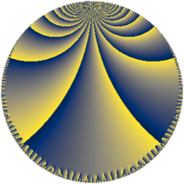# Properties

 Label 128.8.gLevel $128$ Weight $8$ Character orbit 128.g Rep. character $\chi_{128}(17,\cdot)$ Character field $\Q(\zeta_{8})$ Dimension $108$ Sturm bound $128$

# Related objects

## Defining parameters

 Level: $$N$$ $$=$$ $$128 = 2^{7}$$ Weight: $$k$$ $$=$$ $$8$$ Character orbit: $$[\chi]$$ $$=$$ 128.g (of order $$8$$ and degree $$4$$) Character conductor: $$\operatorname{cond}(\chi)$$ $$=$$ $$32$$ Character field: $$\Q(\zeta_{8})$$ Sturm bound: $$128$$

## Dimensions

The following table gives the dimensions of various subspaces of $$M_{8}(128, [\chi])$$.

Total New Old
Modular forms 464 116 348
Cusp forms 432 108 324
Eisenstein series 32 8 24

## Trace form

 $$108 q + 4 q^{3} - 4 q^{5} + 4 q^{7} - 4 q^{9} + O(q^{10})$$ $$108 q + 4 q^{3} - 4 q^{5} + 4 q^{7} - 4 q^{9} + 4 q^{11} - 4 q^{13} + 4 q^{19} - 4 q^{21} + 143420 q^{23} - 4 q^{25} - 476084 q^{27} - 4 q^{29} + 714992 q^{31} - 8 q^{33} - 816500 q^{35} - 4 q^{37} + 283948 q^{39} - 4 q^{41} - 366180 q^{43} + 8744 q^{45} - 3002072 q^{51} - 1815636 q^{53} + 4191012 q^{55} - 4 q^{57} + 1835940 q^{59} - 4559780 q^{61} - 10001872 q^{63} - 8 q^{65} + 1940684 q^{67} + 9580892 q^{69} + 12348708 q^{71} - 4 q^{73} - 11573008 q^{75} - 11914452 q^{77} + 9565884 q^{83} + 312496 q^{85} - 40789108 q^{87} - 4 q^{89} + 3406996 q^{91} - 8752 q^{93} + 54872008 q^{95} - 8 q^{97} + 9738480 q^{99} + O(q^{100})$$

## Decomposition of $$S_{8}^{\mathrm{new}}(128, [\chi])$$ into newform subspaces

The newforms in this space have not yet been added to the LMFDB.

## Decomposition of $$S_{8}^{\mathrm{old}}(128, [\chi])$$ into lower level spaces

$$S_{8}^{\mathrm{old}}(128, [\chi]) \cong$$ $$S_{8}^{\mathrm{new}}(32, [\chi])$$$$^{\oplus 3}$$